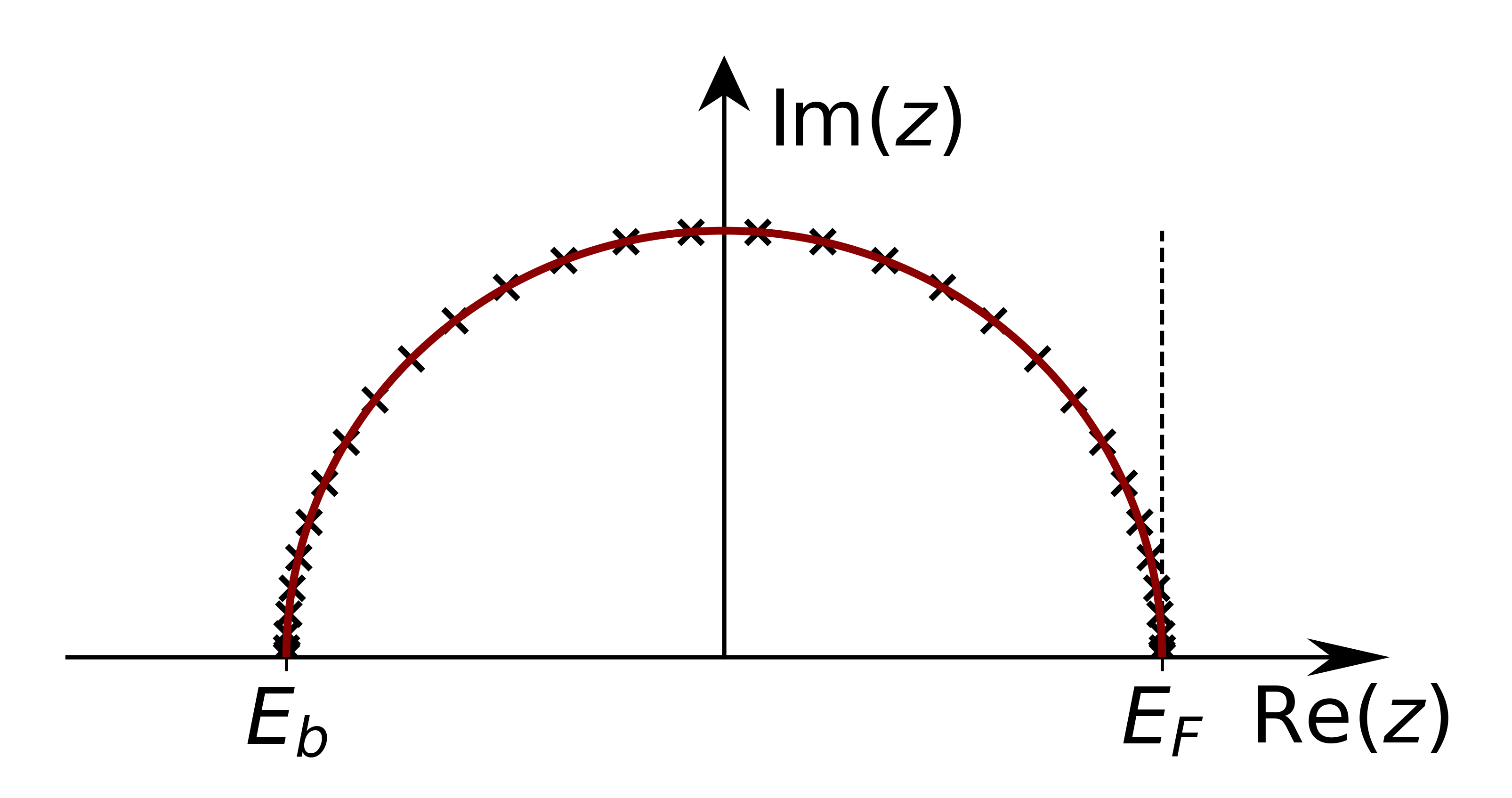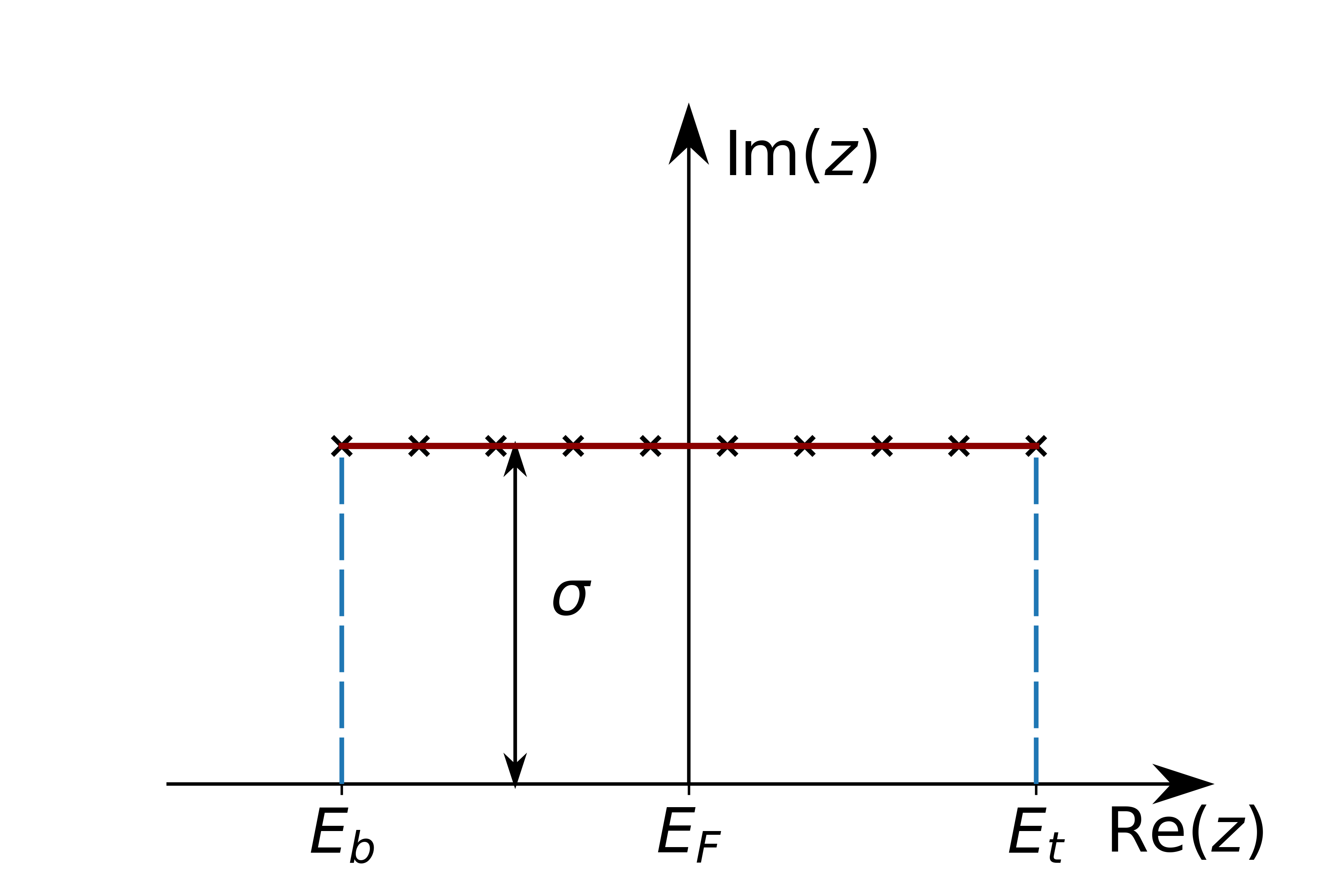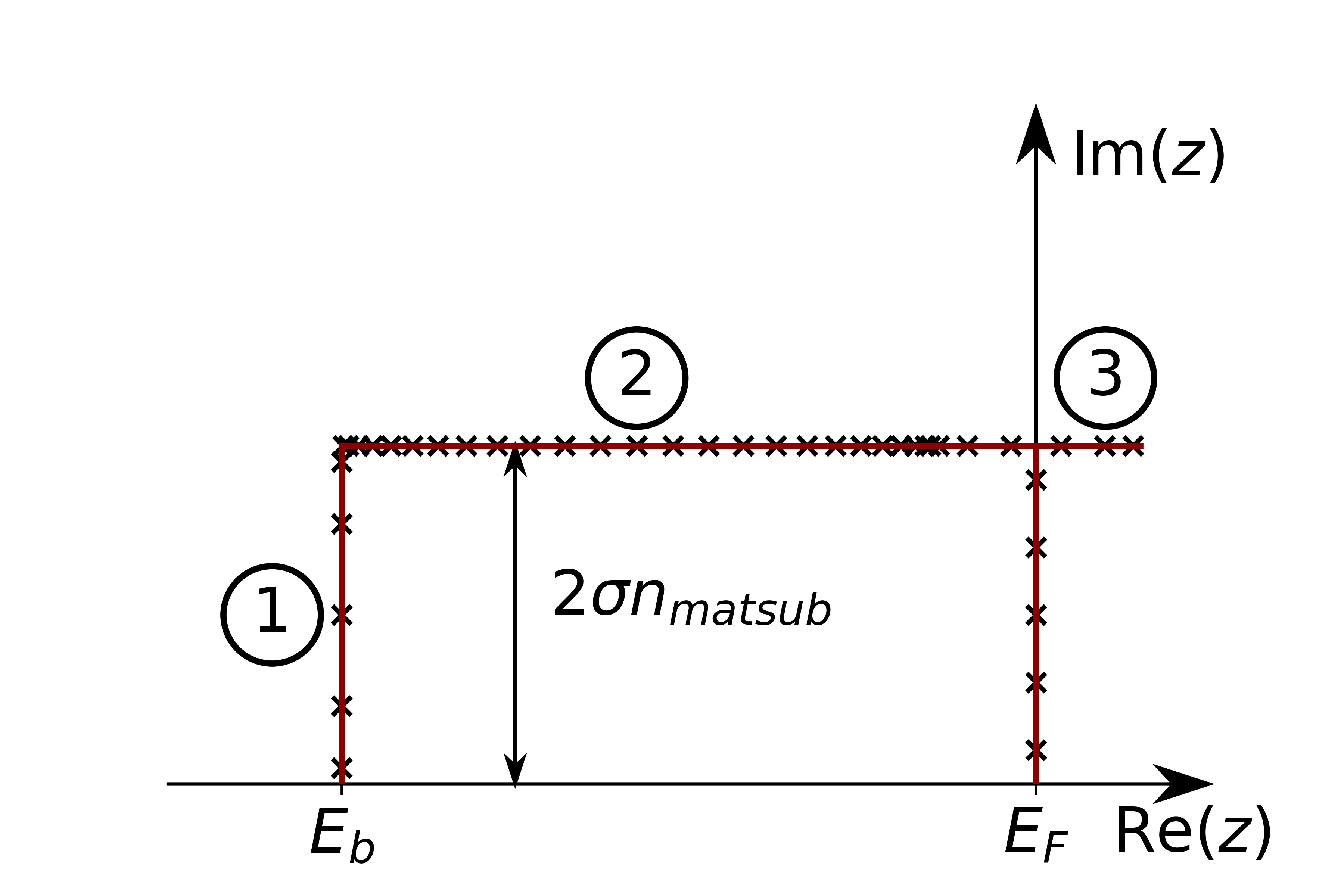### Green's function Setup

#### Energy contour Setup

To calculate a Green's function, the energy contour needs to be defined. This is done by inserting a greensFunction element into the calculationSetup section of the input file. An example is shown here.

    <greensFunction l_mperp="T">
<realAxis ne="5400" ellow="-1.0" elup="1.0"/>
<contourSemicircle n="128" eb="-1.0" et="0.0" alpha="1.0"/>
</greensFunction>


The element has the following attributes:

Attribute Description
l_mperp This logical switch determines whether to calculate the spin-offdiagonal parts in a non-collinear calculation
l_resolvent This optional switch enables the calculation of the resolvent weights for the tetrahedron method (if true). (default: false)
minCalcDistance Defines the charge density distance, below which the Green's Function is calculated. (optional) By default Green's Function is calculated in every iteration
outputSphavg This optional switch will integrate Green's Functions with radial dependence over the Muffin Tin sphere in the output HDF files (if true)
##### Energy Grid

To define the energy grid for the imaginary part of the Green's Function the realAxis element has to be present.

    <realAxis ne="5400" ellow="-1.0" elup="1.0"/>

Attribute Description
ellow Low energy bound of the grid (specified relative to the fermi energy)
elup Upper energy bound of the grid (specified relative to the fermi energy)
##### Complex Energy Contour

For the complex energy contour one or more of the following three elements has to be inserted.

###### Semicircle
    <contourSemicircle n="128" eb="-1.0" et="0.0" alpha="1.0"/>

Attribute Description
eb Low energy bound of the contour (specified relative to the fermi energy)
et Upper energy bound of the contour (specified relative to the fermi energy) (optional: default 0.0)
alpha Factor by which to multiply the imaginary part of the semicircle
label Name of the contour (optional) default:"default"

Defines a semicircle between eb and etSemicircular contour. The points are chosen according to Gauss quadrature.

###### Equidistant Contour
    <contourDOS n="5400" eb="-1.0" et="0.0" sigma="0.001" l_fermi="T" analytical_cont="T"/>

Attribute Description
eb Low energy bound of the contour (specified relative to the fermi energy)
et Upper energy bound of the contour (specified relative to the fermi energy)
sigma Imaginary part for the contour
l_fermi Switch, which determines whether to weight the points according to the Fermi Distribution (if true) or not
analytical_cont Switch, which determines whether to use analytical continuation at the edges of the contour
label Name of the contour (optional) default:"default"

Defines a equdistant contour between eb and et shifted by sigma into the complex plane.Equidistant contour between eb and et. Lines at the edges symbolize the analytical continuation, which can be performed.

###### Rectangular Contour
    <contourRectangle n1="10" n2="128" n3="30" nmatsub="5" eb="-1.0" sigma="0.005"/>

Attribute Description
nmatsub Number of matsubara frequencies considered
eb Low energy bound of the contour (specified relative to the fermi energy)
sigma Defines the inverse temperature to determine the matsubara frequencies
label Name of the contour (optional) default:"default"

Defines a rectangular contour between eb and the Fermi energyRectangular contour. Numbers correspond to the number arguments above. The points in all sections are chosen with Gauss quadrature. At the Fermi Energy the matsubara frequencies are also marked

#### Element Setup

The specification of the Green's Function elements can be provided in another greensfCalculation tag. This element is inserted in the corresponding atomSpecies section behind the energyParameters tag and any ldaU or ldaHIA tags and before the specification of the lo tags.

    <greensfCalculation l_sphavg="T" nshells="0" kkintgrCutoff="calc" label="default">
<!-- here the actual orbital elements are specified (example,details below)/!-->
<diagElements s="F" p="F" d="T" f="T"/>
</greensfCalculation>

Attribute Description
l_sphavg This switch determines, whether the Green's Function is calculated with radial dependence (if false) or not
nshells If this optional argument is not zero the intersite elements with the given number of neighbour shells is calculated
kkintgrCutoff This optional argument specifies how the integration cutoff for the Kramers-Kronig-Transformation is calculated. Default is "calc" -> automatic calculation. For more details see below
label Specifies the energy contour used. Has to correspond to a label in the energy contour setup. default: "default"

If the Green's Functions are supposed to be used for a special calculation, like e.g. the torgue, the format of the element does not change but it is named differently. They may also impose special restrictions on the elements

Valid Name Restrictions
greensfCalculation None
torgueCalculation nshells and l_sphavg arguments are not valid. They are enforced to be 0 and F respectively
##### Specifying the integration cutoff

For the Kramers-Kronig-Transformation it is crucial to define an upper energy cutoff. This can be controlled via the kkintgrCutoff attribute in the greensfCalculation tag. This allows the following arguments.

Attribute Description
'calc' (default) The integration cutoff is automatically determined from the calculated imaginary part. The cutoff is chosen, so that the integration over the DOS derived from the imaginary part is equal to the number of states in the orbital. This only works for onsite and l-diagonal elements
Real Number Specifies the cutoff fixed relative to the Fermi energy
's', 'p', 'd' or 'f' Takes the cutoff for the given l-diagonal element (either specified via 'calc' or a fixed cutoff) and uses it for all elements specified in this tag
##### Selecting the orbital elements

To specify the orbital elements one of the following two elements has to be added in place of the comment above.

Either the l-diagonal elements can be specified

    <diagElements s="F" p="F" d="T" f="T"/>


or a more general definition can be placed here.

    <matrixElements>
<s> F F F F </s>
<p> F F F F </p>
<d> F F T T </d>
<f> F F T T </f>
</matrixElements>


The s, p, d and f tags refer to the orbital quantum numbers, which are allowed for the Green's function calculations.